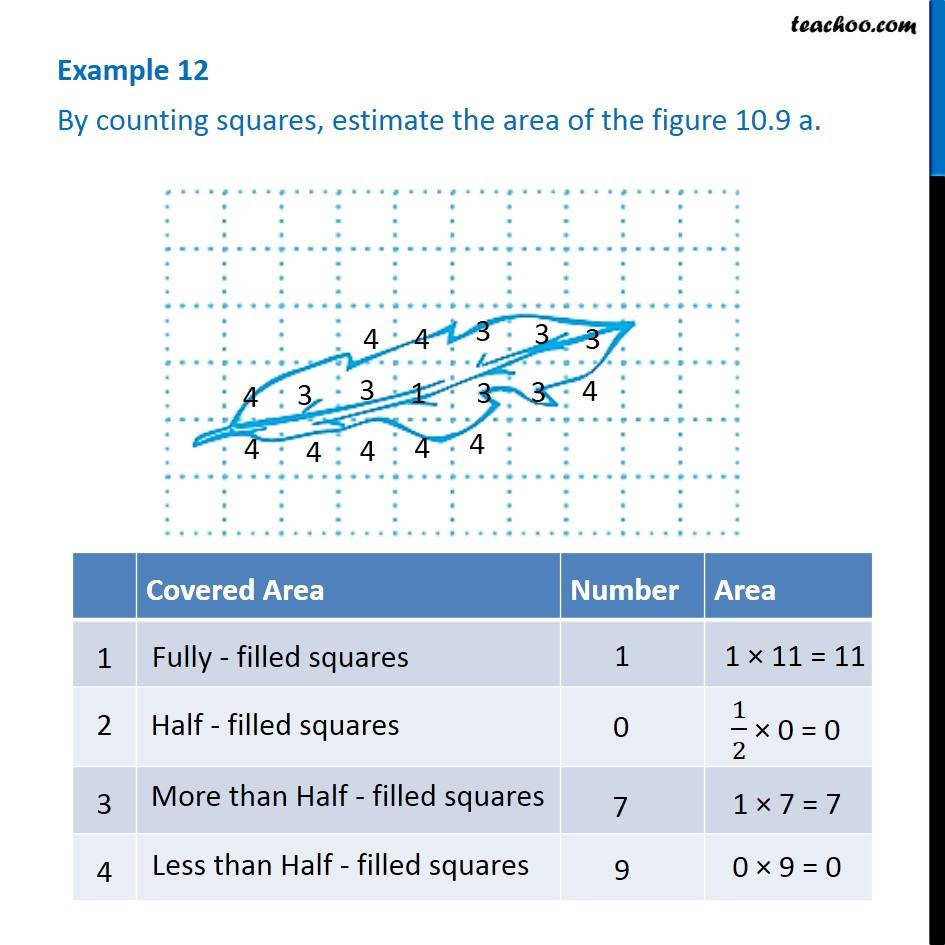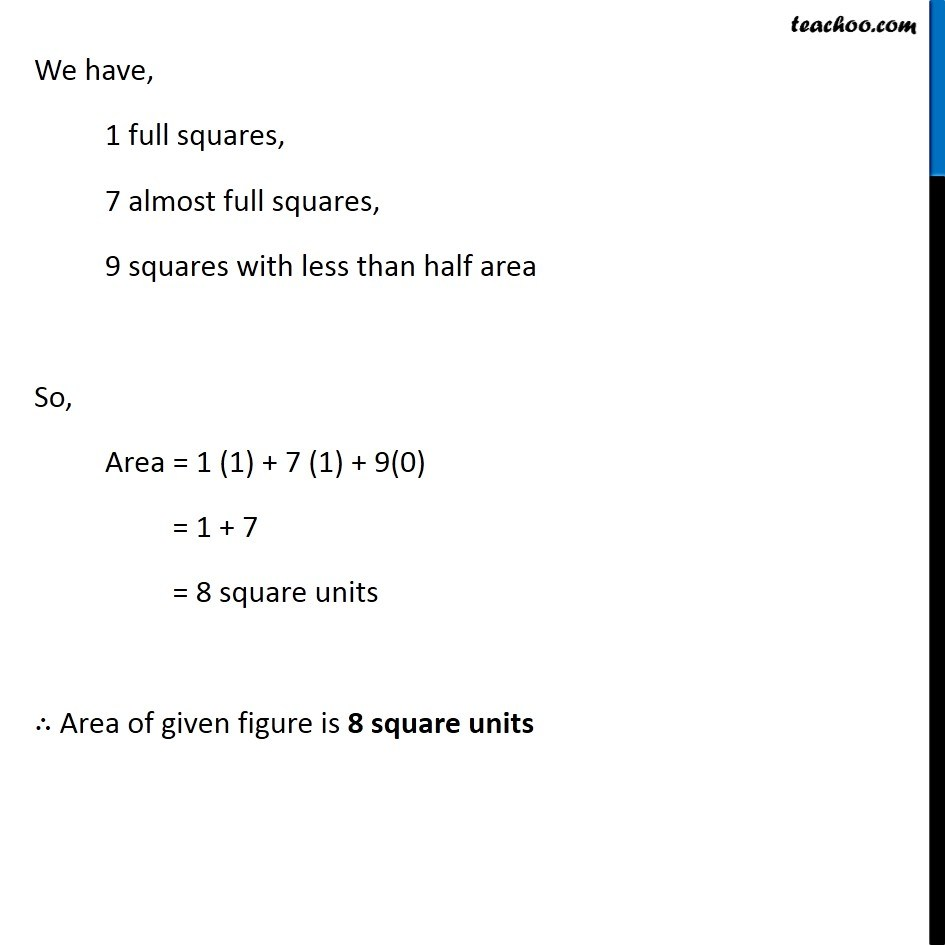1. Chapter 10 Class 6 Mensuration
2. Concept wise
3. Finding area by counting squares

Transcript

Example 12 By counting squares, estimate the area of the figure 10.9 a. We have, 1 full squares, 7 almost full squares, 9 squares with less than half area So, Area = 1 (1) + 7 (1) + 9(0) = 1 + 7 = 8 square units ∴ Area of given figure is 8 square units Example 13 Find the area of a rectangle whose length and breadth are 12 cm and 4 cm respectively Length of rectangle = l = 12 cm Breadth of rectangle = b = 4 cm Area of rectangle = Length × Breadth = 12 × 4 = 48 sq. cm = 48 cm2